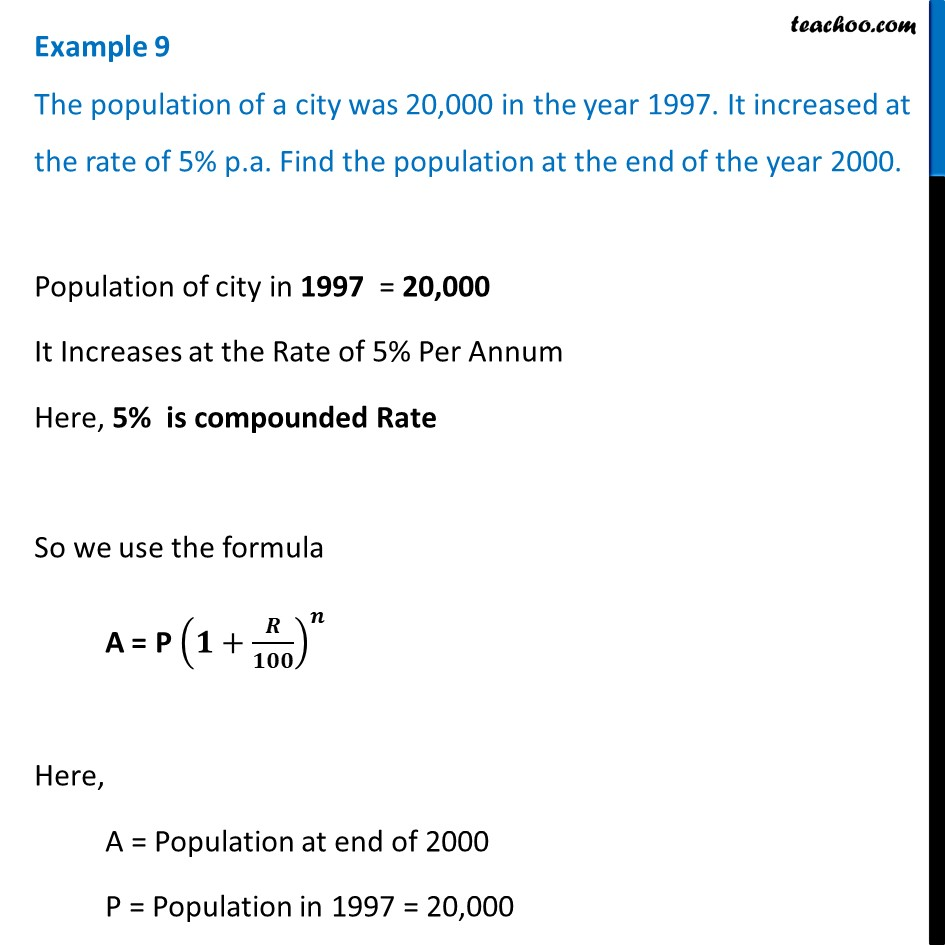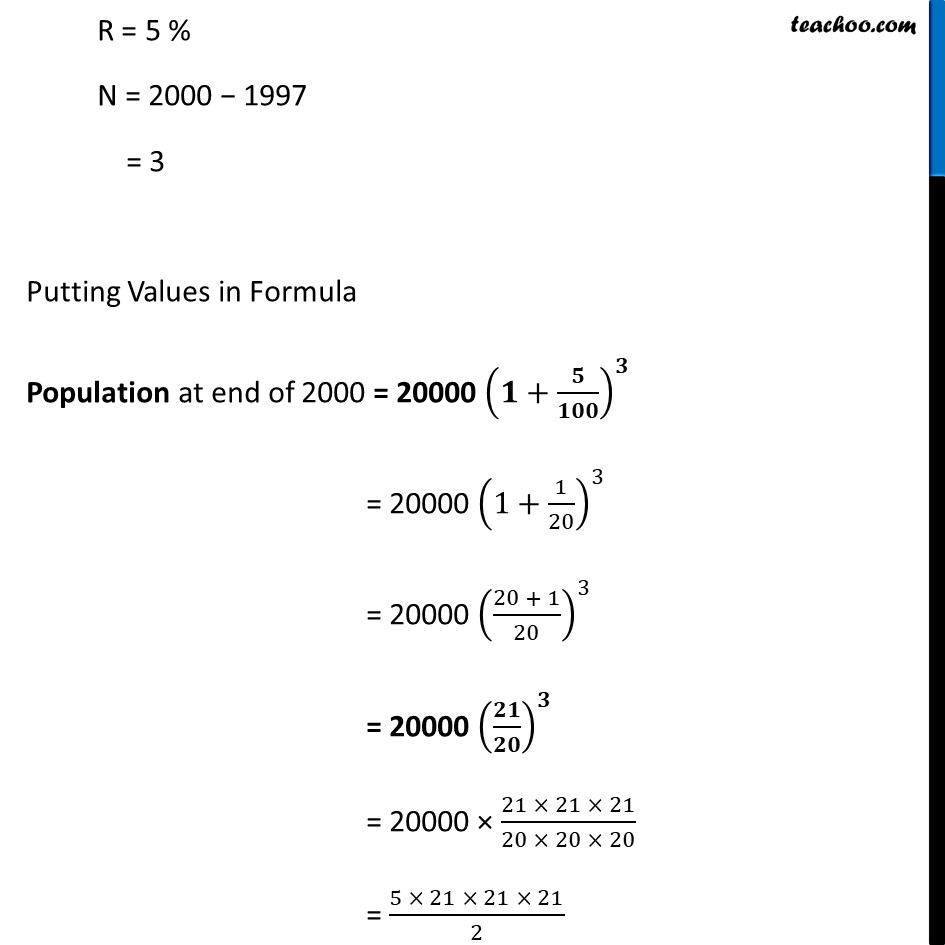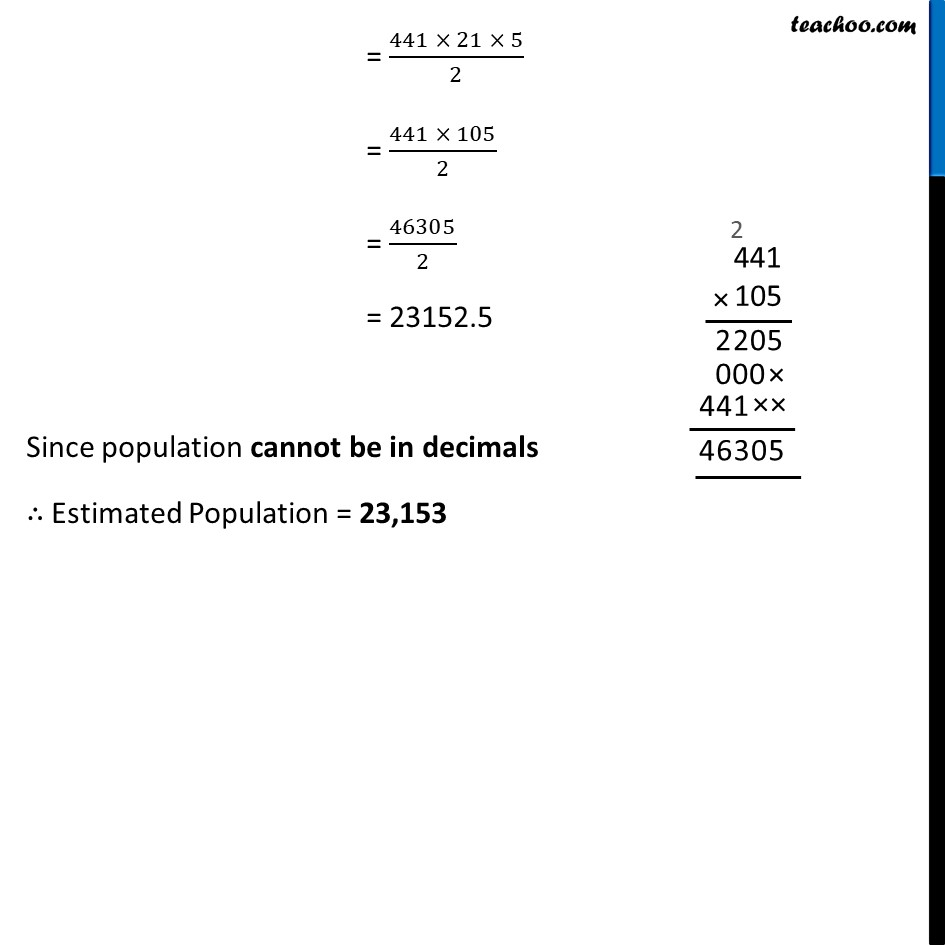Examples

Chapter 7 Class 8 Comparing Quantities
Serial order wiseLearn in your speed, with individual attention - Teachoo Maths 1-on-1 Class

### Transcript

Example 9 The population of a city was 20,000 in the year 1997. It increased at the rate of 5% p.a. Find the population at the end of the year 2000.Population of city in 1997 = 20,000 It Increases at the Rate of 5% Per Annum Here, 5% is compounded Rate So we use the formula A = P (𝟏+𝑹/𝟏𝟎𝟎)^𝒏 Here, A = Population at end of 2000 P = Population in 1997 = 20,000 R = 5 % N = 2000 − 1997 = 3 Putting Values in Formula Population at end of 2000 = 20000 (𝟏+𝟓/𝟏𝟎𝟎)^𝟑 = 20000 (1+1/20)^3 = 20000 ((20 + 1)/20)^3 = 20000 (𝟐𝟏/𝟐𝟎)^𝟑 = 20000 × (21 × 21 × 21)/(20 × 20 × 20) = (5 × 21 × 21 × 21)/2 = (441 × 21 × 5)/2 = (441 × 105)/2 = 46305/2 = 23152.5 Since population cannot be in decimals ∴ Estimated Population = 23,153